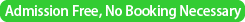### 第8回 統計的機械学習セミナー／The 8th Statistical Machine Learning Seminar

Date
July 12, 2012 (Thursday)Time
13:30-17:45
Place
Seminar room 5,
The Institute of Statistical Mathematics
Speaker 1
Arthur Gretton
(Gatsby Computational Neuroscience Unit, University College London, UK)
Title
Consistent Nonparametric Tests of Independence:
L1, Log-Likelihood and Kernel
Abstract

Three simple and explicit procedures for testing the independence of two multi-dimensional random variables are described. Two of the associated test statistics (L1, log-likelihood) are defined when the empirical distribution of the variables is restricted to finite partitions. A third test statistic is defined as a kernel-based independence measure. Two kinds of tests are provided.

Distribution-free strong consistent tests are derived on the basis of large deviation bounds on the test statistics: these tests make almost surely no Type I or Type II error after a random sample size.

Asymptotically alpha-level tests are obtained from the limiting distribution of the test statistics. For the latter tests, the Type I error converges to a fixed non-zero value alpha, and the Type II error drops to zero, for increasing sample size. All tests reject the null hypothesis of independence if the test statistics become large. The performance of the tests is evaluated experimentally on benchmark data.

Speaker 2
Subhajit Dutta (Indian Statistical Institute, India)
Title
Classification using Localized Spatial Depth with Multiple Localization
Abstract

In the recent past, data depth has been considered by several authors as an effective methodology for supervised and unsupervised classification problems. However, most of the depth based classifiers studied in the literature require the population distributions to be elliptic and unimodal differing only in their locations in order to have satisfactory performance.

Another limitation of such classifiers is that they usually require equal prior probabilities for the populations. Further, for many choices of the well-known depth function, practical implementation of depth based classifiers becomes computationally prohibitive even for moderately large dimensional data. In this talk, we propose a new classifier based on spatial depth, which can be used for high-dimensional. The main idea behind the construction of the proposed classifier is based on fitting generalized additive models to the posterior probabilities corresponding to different classes. In order to cope with possible multimodal and/or non-elliptic nature of the population distributions, we develop a localized version of spatial depth and use that with varying degrees of localization to build the classifier. Our classifier is formed by aggregation of several classifiers each of which is based on spatial depth with a fixed level of localization.

This new classifier can be conveniently used for high-dimensional data, and its possess good discriminatory power for such data. Using some real benchmark data sets, the proposed classifier is shown to have competitive performance when compared with well-known and widely used classifiers like those based on nearest-neighbors, kernel density estimates, support vector machines, classification trees, artificial neural nets, etc.
(This is a joint work with Prof. P. Chaudhuri and Dr. A. K. Ghosh)

Speaker 3
Su-Yun Huang (Institute of Statistical Science, Academia Sinica, Taiwan)
Title
Multilinear Principal Component Analysis -Asymptotic Theory
Abstract

Principal component analysis is commonly used for dimension reduction in analyzing high dimensional data. Multilinear principal component analysis aims to serve a similar function for analyzing tensor structure data, and has empirically been shown effective in reducing dimensionality. In this paper, we investigate its statistical properties and demonstrate its advantages. Conventional principal component analysis, which vectorizes the tensor data, may lead to inefficient and unstable prediction due to the often extremely large dimensionality involved. Multilinear principal component analysis, in trying to preserve the data structure, searches for low-dimensional projections and, thereby, decreases dimensionality more efficiently.

Asymptotic theory of order-two multilinear principal component analysis, including asymptotic efficiency and distributions of principal components, associated projections, and the explained variance, is developed. A test of dimensionality is also proposed.

Finally, multilinear principal component analysis is shown to improve conventional principal component analysis in analyzing the Olivetti faces data set, which is achieved by extracting a more modularly-oriented basis in reconstructing test faces.
(joint with Hung Hung, Pei-Shien Wu and I-Ping Tu)

Speaker 4
Hung Hung
(Institute of Epidemiology and Preventive Medicine, National Taiwan University, Taiwan)
Title
Matrix Variate Logistic Regression Model with Application to EEG Data
Abstract

Logistic regression has been widely applied in the field of biomedical research for a long time. In some applications, covariates of interest have a natural structure, such as being a matrix, at the time of collection. The rows and columns of the covariate matrix then have certain physical meanings, and they must contain useful information regarding the response.

If we simply stack the covariate matrix as a vector and fit a conventional logistic regression model, relevant information can be lost, and the problem of inefficiency will arise. Motivated from these reasons, we propose in this paper the matrix variate logistic (MV-logistic) regression model. Advantages of MV-logistic regression model include the preservation of the inherent matrix structure of covariates and the parsimony of parameters needed. In the EEG Database Data Set, we successfully extract the structural effects of covariate matrix, and a high classification accuracy is achieved. (Joint with Chen-Chien Wang) MV-logistic regression belongs to the class of tensor regression, which now attracts the attention of statisticians. In this talk, I will also introduce some our recent developments of tensor regression, and will focus on its application to the detection of gene-gene interactions.

▲ このページのトップへ